# Six-digit primes

Find all six-digit prime numbers that contain each one of digits 1,2,4,5,7 and 8 just once. How many are they?

Correct result:

n =  0

#### Solution:

$n=0$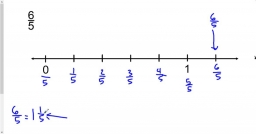We would be pleased if you find an error in the word problem, spelling mistakes, or inaccuracies and send it to us. Thank you!## Next similar math problems:

• Prime factorsWrite 98 as product of prime factors
• Primes 2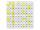Which prime numbers is number 2025 divisible?
• Divisibility 2How many divisors has integer number 13?
• Sum of two primesChristian Goldbach, a mathematician, found out that every even number greater than 2 can be expressed as a sum of two prime numbers. Write or express 2018 as a sum of two prime numbers.
• Two-digit numberI am a two-digit number less than 20. When I divided by three, then you get the rest 1 and when you divide me by four you get also rest 1. What number am I?
• Digit sumDetermine for how many integers greater than 900 and less than 1,001 has digit sum digit of the digit sum number 1.
• Dance ensemble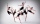The dance ensemble took the stage in pairs. During dancing, the dancers gradually formed groups of four, six and nine. How many dancers have an ensemble?
• Toy carsPavel has a collection of toy cars. He wanted to regroup them. But in the division of three, four, six, and eight, he was always one left. Only when he formed groups of seven, he divided everyone. How many toy cars have in the collection?
• NumberWhat number should be placed instead of the asterisk in number 702*8 to get a number divisible by 6?
• MO Z6-6-1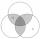Write integers greater than 1 to the blanks in the following figure, so that each darker box was product of the numbers in the neighboring lighter boxes. What number is in the middle box?
• LCM of two numberFind the smallest multiple of 63 and 147
• Bikes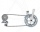Pepa asked Honza how many bikes he had. He replied that he had three-quarters of their total number and another three-quarter of one bike. So how many bikes does Honza have?
• Year 2018The product of the three positive numbers is 2018. What are the numbers?
• MO C–I–1 2018An unknown number is divisible by just four numbers from the set {6, 15, 20, 21, 70}. Determine which ones.
• Unknown numberUnknown number is divisible by exactly three different primes. When we compare these primes in ascending order, the following applies: • Difference first and second prime number is half the difference between the third and second prime numbers. • The prod
• One hundred stamps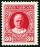A hundred letter stamps cost a hundred crowns. Its costs are four levels - twenty tenths , one crown, two-crown and five-crown. How many are each type of stamps? How many does the problem have solutions?
• Z9–I–4 MO 2017Numbers 1, 2, 3, 4, 5, 6, 7, 8 and 9 were prepared for a train journey with three wagons. They wanted to sit out so that three numbers were seated in each carriage and the largest of each of the three was equal to the sum of the remaining two. The conduct